## Main

Several teams have engineered detectors that are sensitive to single microwave photons by strongly coupling atoms (or qubits) to high-quality-factor (high-Q) cavities. This architecture, known as cavity quantum electrodynamics (cavity QED), can be used in various ways to detect photons. One destructive method measures quantum Rabi oscillations of an atom or qubit resonantly coupled to the cavity8,9,10. The oscillation frequency is proportional to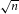, where n is the number of photons in the cavity, so this method essentially measures the time-domain swap frequency.

Another method uses a dispersive interaction to map the photon number in the cavity onto the phase difference of a superposition of atomic states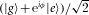. Each photon number n corresponds to a different phase φ, so repeated Ramsey experiments5 can be used to estimate the phase and extract n. This method is QND, because it does not exchange energy between the atom and photon. However, as the phase cannot be measured in a single operation, it does not extract full information about a particular Fock state |n〉 in a single interrogation. Nonetheless, using Rydberg atoms in cavity QED, remarkable experiments have shown quantum jumps of light and the collapse of the photon number by measurement5,12.

Here we report a new method that implements a set of programmable controlled-NOT (CNOT) operations between an n-photon Fock state and a qubit, asking the question ‘are there exactly n photons in the cavity?’ A single interrogation consists of applying one such CNOT operation and reading-out the resulting qubit state. To do this we use a quasi-dispersive qubit–photon interaction that causes the qubit transition frequency to depend strongly on the number of photons in the cavity. Consequently, frequency control of a pulse implements a conditional π rotation on the qubit—the qubit state is inverted if and only if there are n photons in the storage cavity. To ensure that this is QND, the qubit and storage cavity are adiabatically decoupled before carrying out a measurement of the qubit state.

To realize this method we extend circuit-based cavity QED (ref. 13) by coupling a single transmon qubit14,15 simultaneously to two cavities. In this scheme, the transmon qubit is used to interrogate the state of one cavity; then the transmon is measured with the other cavity. This separation of functions allows one cavity to be optimized for coherent storage of photons (high-Q) and the other for fast qubit readout (low-Q). Related work by Leek et al. 16 realized a single transmon coupled to two modes of a single cavity, where the two modes were engineered to have very different quality factors. A schematic of the two-cavity device is shown in Fig. 1a. The cavities are realized as Nb coplanar waveguide resonators with λ/2 resonances at ωs/2π=5.07 GHz and ωm/2π=6.65 GHz, respectively. The cavities are engineered, by design of the capacitors Cs and Cm, to have very different decay rates (κs/2π=50 kHz and κm/2π=20 MHz) so that the qubit state can be measured several times per photon lifetime in the storage cavity. By having these cavities at different frequencies, the fast decay of the readout cavity does not adversely affect photons in the storage cavity. A transmon qubit is end-coupled to the two cavities, with finger capacitors controlling the individual coupling strengths (gs/2π=70 MHz and gm/2π=83 MHz). The usual shunt capacitor between the transmon islands is replaced with capacitors to the ground planes to reduce direct coupling between the cavities. Furthermore, a flux bias line17 allows fast, local control of the magnetic field near the transmon. This facilitates manipulations of the detunings Δs=ωg,eωs and Δm=ωg,eωm between the transmon and cavities, where we use the convention of labelling the transmon states from lowest to highest energy as (g,e,f,h,…).

To achieve high photon-number selectivity of the CNOT operations, there must be a large separation between the number-dependent qubit transition frequencies. To obtain this, we use small detunings (Δs/gs<10) between the qubit and storage cavity. Figure 2 shows spectroscopy in this quasi-dispersive regime as a function of flux bias when the storage cavity is populated with a coherent state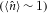. A numerical energy-level calculation is overlaid, showing the positions of various transitions. We define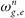as the photon-number-dependent transition frequency |n,g〉→|n,e〉. Other transitions, such as |2,g〉→|0,h〉, are allowed because of the small detuning. Fortunately, we also see that the separation between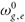and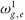grows rapidly to order 2 gs=140 MHz as the qubit approaches the storage cavity.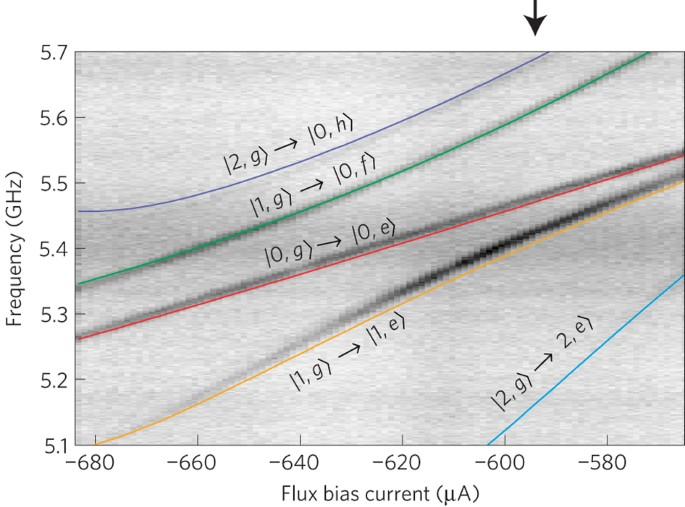Figure 2: Pulsed spectroscopy with a coherent state in storage cavity (〈n〉≈1) versus qubit–cavity detuning Δs=ωg,e−ωs.

To test the photon meter, we generate single photons in the storage cavity with an adiabatic protocol. Our method uses the avoided crossing between the |0,e〉 and |1,g〉 levels to convert a qubit excitation into a photon. The preparation of a photon begins with the qubit detuned below the storage cavity (Δs−3gs), where we apply a π-pulse to create the state |0,e〉. We then adiabatically tune the qubit frequency through the avoided crossing with the storage cavity, leaving the system in the state |1,g〉. The sweep rate is limited by Landau–Zener transitions that keep the system in |0,e〉. The preparation protocol changes the qubit frequency by 600 MHz in 50 ns, giving a spurious transition probability less than 0.1% (calculated with a multi-level numerical simulation). This protocol actually allows for the creation of arbitrary superpositions of |0,g〉 and |1,g〉 by changing the rotation angle of the initial pulse. For example, if we use a π/2-pulse, after the sweep the system ends up in the state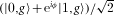, where φ is determined by the rotation axis of the π/2-pulse. One could also use a resonant swap scheme, which has been successfully used to create Fock states9 up to |n=15〉. The method used here has the advantage of being very robust to timing errors.

After the photon is prepared in the storage cavity, the qubit frequency is adjusted such that Δs/gs5. At this detuning, the separation betweenandis 65 MHz. In Fig. 3a, we show pulsed spectroscopy at this detuning for several rotation angles of the initial preparation pulse. We observe well-resolved dips in the reflected phase of a pulsed signal sent at the measurement cavity frequency. The locations of these dips correspond to the qubit transition frequencies for n=0 () and n=1 (), and the relative heights match expectations from the different preparation-pulse rotations (for example, a π/2-pulse results in equal-height signals).

To show selective driving of these transitions, we carry out Rabi experiments atandfor the cases where we prepare |0,g〉 and |1,g〉. In each experiment we ensemble average measurements of the resulting qubit state after further decoupling the qubit from the storage cavity. For the |0,g〉 case (Fig. 3b) there is a large-amplitude oscillation when the drive is at(red, R0(θ)) and almost no oscillation when the drive is at(blue, R1(θ)). When we prepare |1,g〉 the situation is reversed (Fig. 3c); however, in this case the residual oscillation of R0(θ) (red) is substantial because of small errors in the preparation of |1,g〉 associated with the initial rotation of the qubit and, more importantly, the 10% probability of energy decay during the subsequent adiabatic sweep through the cavity.

The responses Ri(θ) are a result of driving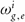and the far off-resonant drive of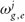, where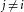. The cross-talk is seen in the small residual oscillation of R1(θ) in Fig. 3b. In the Supplementary Information, we derive a method for extracting a selectivity and preparation fidelity from these data, giving a selectivity ≥95% for both interrogations and a preparation fidelity of |〈n=1|ψ〉|2≈88%. These numbers were confirmed by doing equivalent experiments over a range of preparation-pulse rotation angles between 0 and 2π (not shown).

If π-pulses are used in the interrogation step, measurement results of the average qubit state directly correlate with the probability of being in the states |n=0〉 or |n=1〉. Details of the scaling needed to do this transformation when the selectivity is <100% are presented in the Supplementary Information. These are the desired CNOT operations of the photon meter. If we now insert a variable delay before interrogating, we find that P0 (P1), the probability of being in |n=0〉 (|n=1〉), decays exponentially towards 1 (0), as shown by the red (orange) trace in Fig. 4b. The decay constant of T13.11±0.02 μs agrees with the linewidth of the storage cavity, 1/κs=1/(2π 50 kHz)=3.2±0.1 μs, measured in a separate, low-power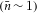reflection experiment.